Question

The historical returns on a portfolio had an average return of 24 percent and a standard deviation of 31 percent. Assume that returns on this portfolio follow a bell-shaped distribution.

a.
Approximately what percentage of returns were greater than 86 percent? (Round your answer to the nearest whole percent.)

b.
Approximately what percentage of returns were below –69 percent? (Round your answer to 1 decimal place.)

Solution :

Given that ,

mean =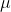= 24% = 0.24

standard deviation =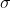= 31% = 0.31

(a) 86% = 0.86

P(x > 0.86) = 1 - P(x < 0.86)

= 1 - P((x -) /< (0.86 - 0.24) / 0.31)

= 1 - P(z < 0.02)

= 1 - 0.508

= 0.492

(b)

-0.69% = -0.69

P(x < -0.69)

= P((x -) /< (-0.69 - 0.24) / 0.31)

= P(z < -3)

= 0.0013

= 0.1%

#### Earn Coins

Coins can be redeemed for fabulous gifts.

Similar Homework Help Questions
• ### The historical returns on a portfolio had an average return of 15 percent and a standard...

The historical returns on a portfolio had an average return of 15 percent and a standard deviation of 18 percent. Assume that returns on this portfolio follow a bell-shaped distribution. a. Approximately what percentage of returns were greater than 33 percent? (Round your answer to the nearest whole percent.) Percentage of returns b. Approximately what percentage of returns were below –21 percent? (Round your answer to 1 decimal place.) Percentage of returns

• ### The historical returns on a portfolio had an average return of 21 percent and a standard...

The historical returns on a portfolio had an average return of 21 percent and a standard deviation of 29 percent. Assume that returns on this portfolio follow a bell-shaped distribution. a. Approximately what percentage of returns were greater than 79 percent? (Round your answer to the nearest whole percent.) b. Approximately what percentage of returns were below –66 percent? (Round your answer to 1 decimal place.) ____________________________________________________________________________________________________________________________ The following relative frequency distribution was constructed from a population of 400. Calculate...

• ### The historical returns on a balanced portfolio have had an average return of 8% and a...

The historical returns on a balanced portfolio have had an average return of 8% and a standard deviation of 15%. Assume that returns on this portfolio follow a normal distribution. [Use Excel commands instead of the z table.] a. What percentage of returns were greater than 53%? (Round your answer to 2 decimal places.)

• ### 4. The historical returns on a balanced portfolio have had an average return of 8 %...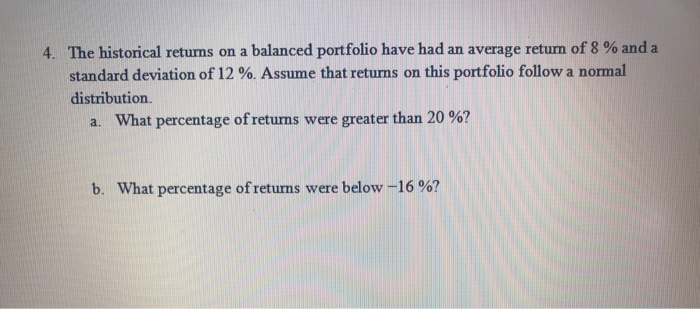4. The historical returns on a balanced portfolio have had an average return of 8 % and a standard deviation of 12 %. Assume that returns on this portfolio follow a normal distribution a. What percentage of returns were greater than 20 %? b. What percentage of returns were below -16 %?

• ### Please provide simple explanation 6 The historical returns on a balanced portfolio have had an average...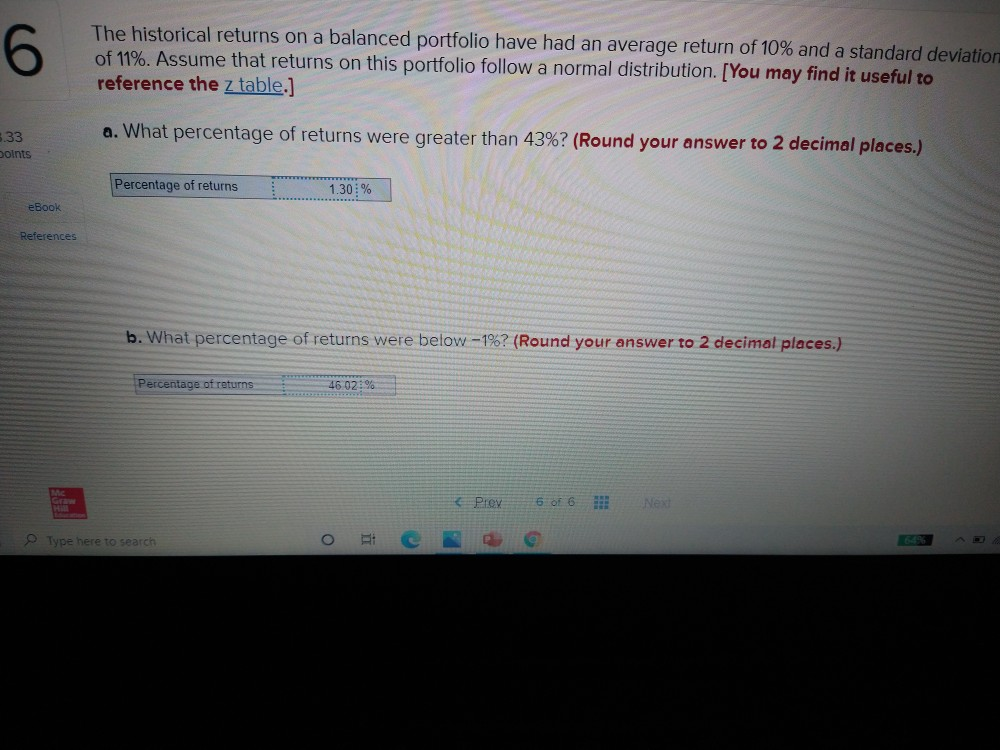Please provide simple explanation 6 The historical returns on a balanced portfolio have had an average return of 10% and a standard deviation of 11%. Assume that returns on this portfolio follow a normal distribution. [You may find it useful to reference the z table.] a. What percentage of returns were greater than 43%? (Round your answer to 2 decimal places.) 5.33 points Percentage of returns 1.30: % eBook References b. What percentage of returns were below -1%? (Round your...

• ### Please provide steps that are simple. Thank you. The historical returns on a balanced portfolio have...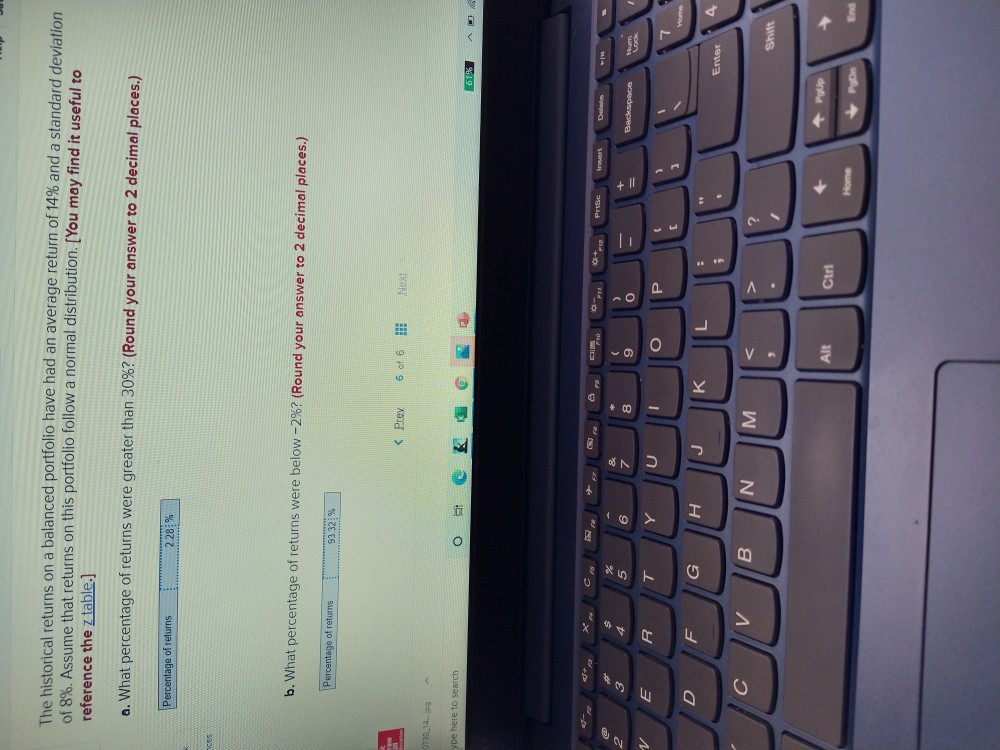Please provide steps that are simple. Thank you. The historical returns on a balanced portfolio have had an average return of 14% and a standard deviation of 8%. Assume that returns on this portfolio follow a normal distribution. [You may find it useful to reference the z table.] a. What percentage of returns were greater than 30%? (Round your answer to 2 decimal places.) Percentage of returns 228% ces b. What percentage of returns were below -2%? (Round your answer...

• ### Data are drawn from a bell-shaped distribution with a mean of 95 and a standard deviation...

Data are drawn from a bell-shaped distribution with a mean of 95 and a standard deviation of 6. a. Approximately what percentage of the observations fall between 83 and 107? (Round your answer to the nearest whole percent.) b. Approximately what percentage of the observations fall between 77 and 113? (Round your answer to the nearest whole percent.) c. Approximately what percentage of the observations are less than 83? (Round your answer to 1 decimal place.)

• ### Data are drawn from a bell-shaped distribution with a mean of 80 and a standard deviation...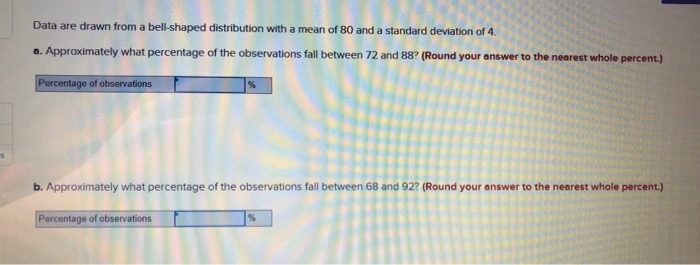Data are drawn from a bell-shaped distribution with a mean of 80 and a standard deviation of 4 a. Approximately what percentage of the observations fall between 72 and 88? (Round your answer to the nearest whole percent.) Percentage of observations b. Approximately what percentage of the observations fall between 68 and 92? (Round your answer to the nearest whole percent.) Percentage of observations c. Approximately what percentage of the observations are less than 76? (Round your answer to 1...

• ### A portfolio has average return of 13.2 percent and standard deviation of returns of 18.9 percent....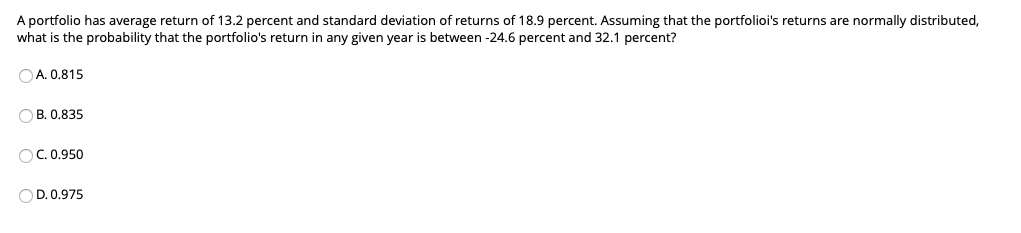A portfolio has average return of 13.2 percent and standard deviation of returns of 18.9 percent. Assuming that the portfolioi's returns are normally distributed, what is the probability that the portfolio's return in any given year is between -24.6 percent and 32.1 percent? A. 0.815 B. 0.835 ос C. 0.950 D. 0.975 A portfolio has expected return of 13.2 percent and standard deviation of 18.9 percent. Assuming that the returns of the portfolio are normally distributed, what is the probability...

• ### Consider a sample with 10 observations of 11, –3, 8, 8, 10, 1, –2, 13, 8,...

Consider a sample with 10 observations of 11, –3, 8, 8, 10, 1, –2, 13, 8, and –4. Use z-scores to determine if there are any outliers in the data; assume a bell-shaped distribution. (Round your answers to 2 decimal places. Negative values should be indicated by a minus sign.)   The z-score for the smallest observation      The z-score for the largest observation      There are outliers or no outliers in the data. The historical returns on a portfolio had...